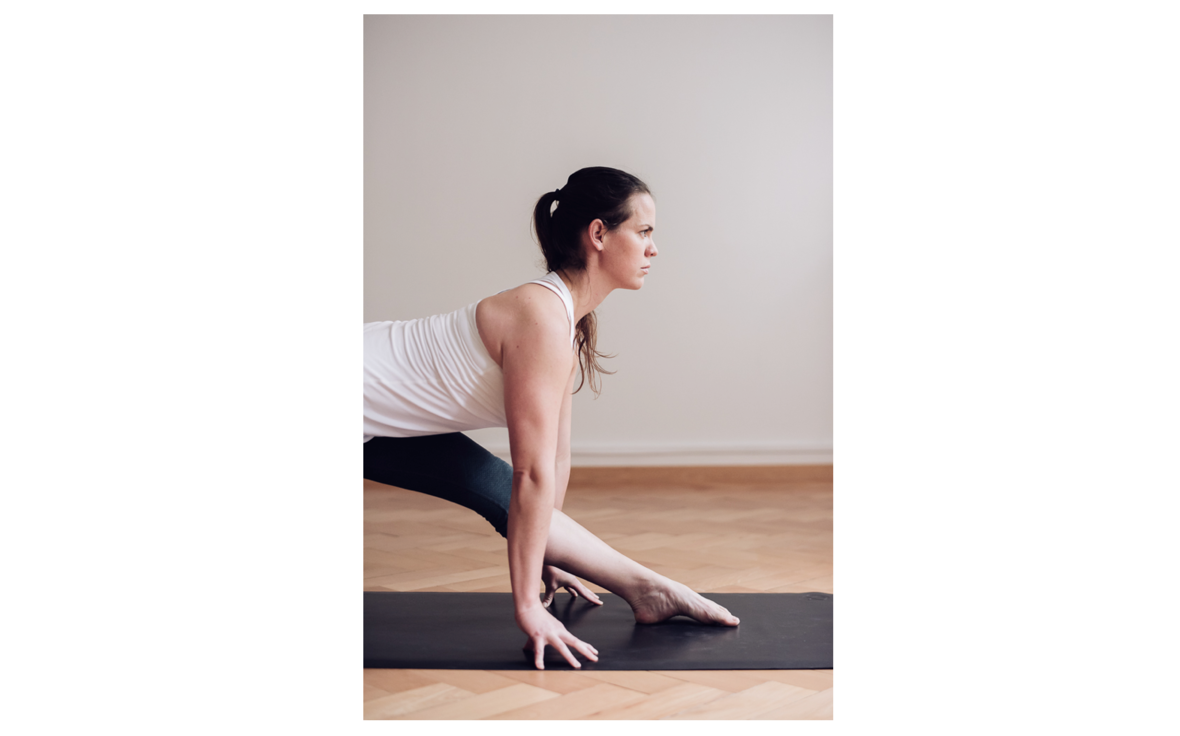## OCTOBER 2018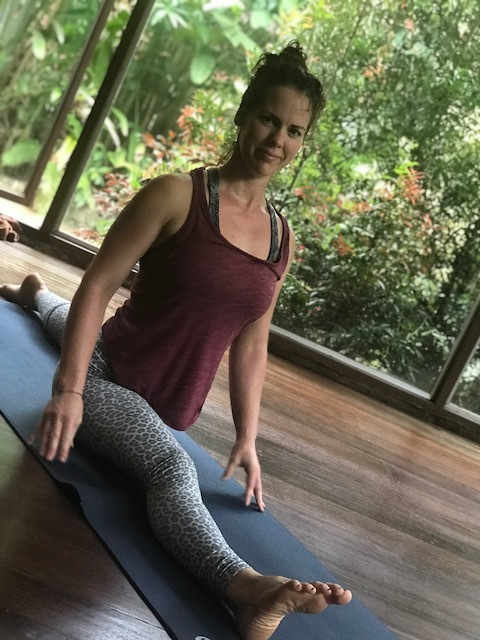What does authenticity mean to you?

## October I Love you so!

It has been a super fast month of September and the transition into the cooler weather last week has been ever so sweet! There is a lot of information for you in this newsletter! Check out the hanuman shala SPECIALS and the 2018 Countdown section!

## Zurich | You've got me Back!

...at least for a little while. This month I will be starting a new Yoga Lunch class at The Space in Zurich! Come on out this Tuesday if you can! Plus, if you haven't signed up for my birthday class on Thursday at Yoga Tribe K3 please do so! We have limited space available!

I am also starting with the Mom + Babe Yoga Course on October 22! Do you know anyone with a new bundle of magic that would love to move and connect! More info on it below!

## Yoga Plans

There will be an extra newsletter again this month regarding the 3 types of trainings that I will be offering. Study with me in 2019! Stay tuned for it!

Yoga in Kilchberg and the Relax + Breathe class are happening every month from now on until the end of 2018! Take advantage of it!

## Next Steps

There is so much more but I have to be honest and say that I am a little bit overworked! Yes, even Yoga Teachers get there sometimes! Even though it might not look like it from the schedule below, I have actually eliminated and cancelled quite a few engagements. That said, I have prioritized my own practice (on and off the mat) and will pull back - next year. More on my steps in the next newsletter... Know that I am here for you and if you want to work on your self with me; keep coming to class.

 table div table+table+table+table+table div table{width:100%;padding:0}table div table+table+table+table+table div table img{width:96.23%;padding:0;float:none}table div table+table+table+table+table div table td{width:100%;padding:0 1.88% 18px}/* styles */Mountain Love

## Intention of the Month

This month ask yourself; What are you holding on to that you no longer need?Our intention in October is to truly look within. Are you holding on to a friendship that is no longer nourishing? Or are you constantly pushing yourself into a frame that doesn't actually fit you? You can also apply this towards materialism and check out how much stuff you have that you actually don't need and never use anyway. Take the month of October to purge, to release and to make space. I will reveal what has worked for me to let go and share a little bit of my own cleansing process soon. If you feel like you want to share your path with me, reach out.

 table div table+table+table+table+table+table+table div table{width:100%;padding:0}table div table+table+table+table+table+table+table div table img{width:96.23%;padding:0;float:none}table div table+table+table+table+table+table+table div table td{width:100%;padding:0 1.88% 18px}/* styles */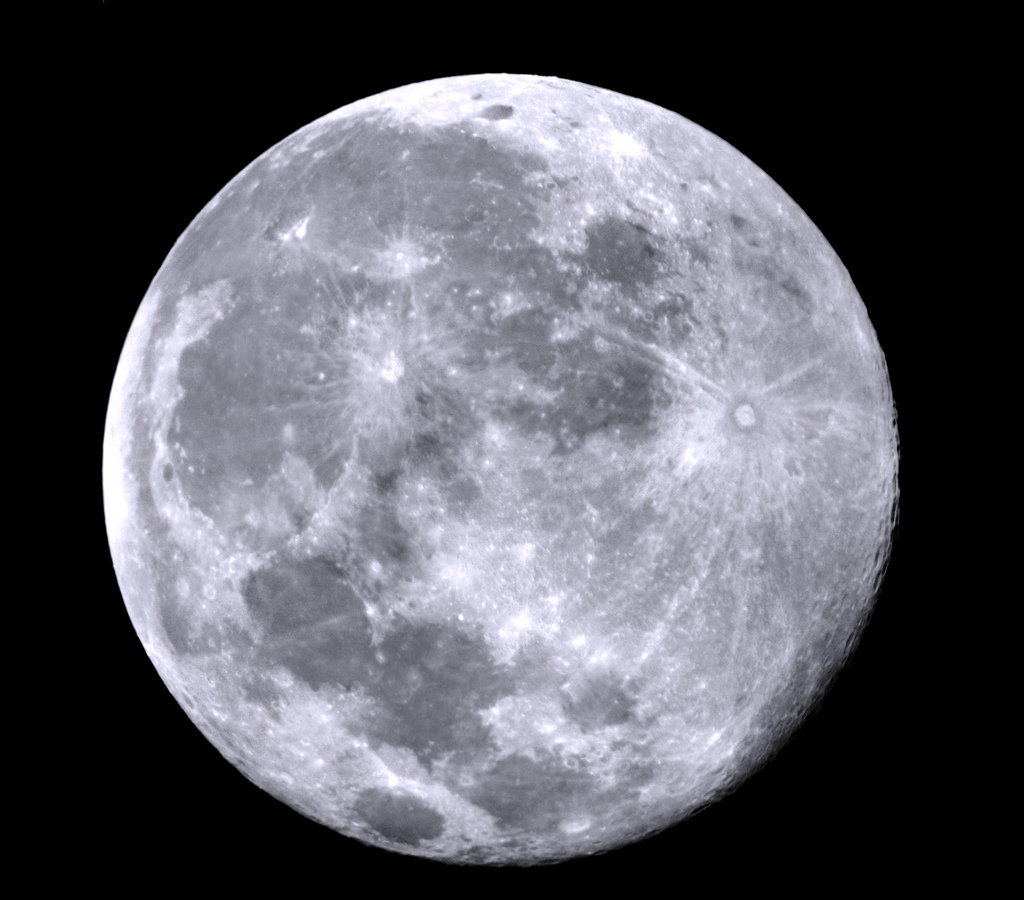An important reminder of change!

## Moon Days

New Moon - Tuesday 9.10 | 05:46

Full Moon - Wednesday 24.10 | 18:45

 table div table+table+table+table+table+table+table+table+table div table{width:100%;padding:0}table div table+table+table+table+table+table+table+table+table div table img{width:96.23%;padding:0;float:none}table div table+table+table+table+table+table+table+table+table div table td{width:100%;padding:0 1.88% 18px}/* styles */## Writing on YogaWiser

I am sharing an older piece, I wrote this about a year ago; The Way it Dies until Birth. It is a bit more on the heavy and dense side but still worth reading. Moving your body through yoga can bring up so much stuff! My teacher Tim Miller likes to say; "Practice and all is coming, the good and the bad." How has your yoga practice been going?
(I promise to bring something lighter next time!)

 table div table+table+table+table+table+table+table+table+table+table+table div table{width:100%;padding:0}table div table+table+table+table+table+table+table+table+table+table+table div table img{width:96.23%;padding:0;float:none}table div table+table+table+table+table+table+table+table+table+table+table div table td{width:100%;padding:0 1.88% 18px}/* styles */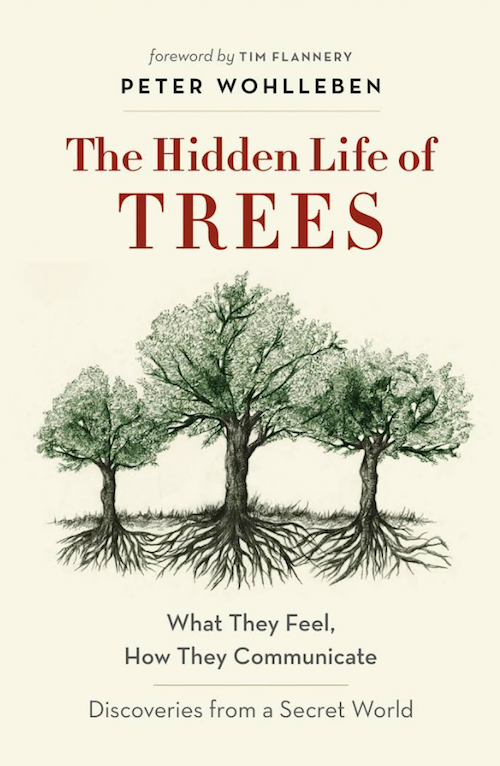## Book Corner

This month I'd like to suggest my newest gem and absolut favorite book at the moment: The Hidden Life of Trees by Peter Wohlleben or in German Das geheime Leben der Bäume. The book is originally written in German. I absolutely love this book! It is a great October read as well! It is rich in information and I love how one can take tree communication into our human communication! Did you ever feel into the energy when we are in a public place, surrounded by people? You can feel if people are busy, scared, loving, etc. can't you? Or think about being in a yoga class with people you know vs. in a random workshop / class where you don't know anyone, not even the teacher. Hence, the energy is very different! If you love the forest and trees this is a must read!

 table div table+table+table+table+table+table+table+table+table+table+table+table+table div table{width:100%;padding:0}table div table+table+table+table+table+table+table+table+table+table+table+table+table div table img{width:96.23%;padding:0;float:none}table div table+table+table+table+table+table+table+table+table+table+table+table+table div table td{width:100%;padding:0 1.88% 18px}/* styles *//* styles */ Have a wonderful OCTOBER month YOGI See you on the mat! Much Love + Peace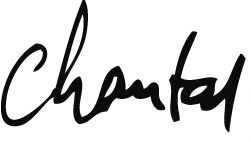## P.S.

I am excited to see you again! It feels like it's been forever!

 table div table+table+table+table+table+table+table+table+table+table+table+table+table+table+table+table+table div table{width:100%;padding:0}table div table+table+table+table+table+table+table+table+table+table+table+table+table+table+table+table+table div table img{width:96.23%;padding:0;float:none}table div table+table+table+table+table+table+table+table+table+table+table+table+table+table+table+table+table div table td{width:100%;padding:0 1.88% 18px}/* styles */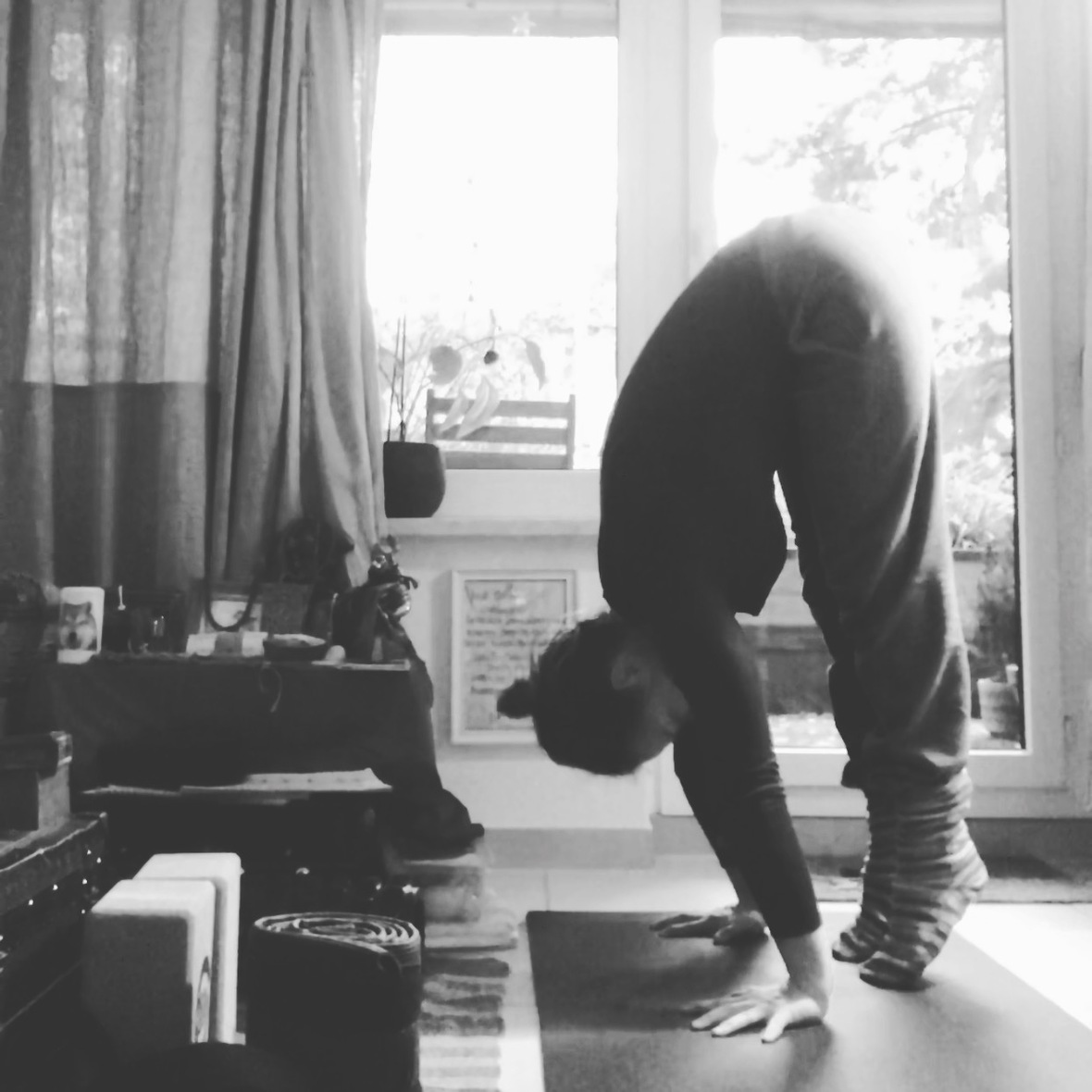table div table+table+table+table+table+table+table+table+table+table+table+table+table+table+table+table+table+table+table+table div table{width:100%;padding:0}table div table+table+table+table+table+table+table+table+table+table+table+table+table+table+table+table+table+table+table+table div table img{width:96.23%;padding:0;float:none}table div table+table+table+table+table+table+table+table+table+table+table+table+table+table+table+table+table+table+table+table div table td{width:100%;padding:0 1.88% 18px}/* styles */## Tuesdays - Dienstags

NEW CLASS!!!
12:15 - 13:15 Hanuman Flow (EN)
at The Space in Zürich | K5

17:30 - 18:45 Basic + Neu (CH)
at Living Yoga in Luzern

19:00 - 20:30 Hanuman Flow (EN)
at Living Yoga in Luzern

 table div table+table+table+table+table+table+table+table+table+table+table+table+table+table+table+table+table+table+table+table+table+table div table{width:100%;padding:0}table div table+table+table+table+table+table+table+table+table+table+table+table+table+table+table+table+table+table+table+table+table+table div table img{width:96.23%;padding:0;float:none}table div table+table+table+table+table+table+table+table+table+table+table+table+table+table+table+table+table+table+table+table+table+table div table td{width:100%;padding:0 1.88% 18px}/* styles */## Wednesdays - Mittwochs

10:00 - 11:30 Hanuman Flow (EN)
at Planet Yoga in Zürich

 table div table+table+table+table+table+table+table+table+table+table+table+table+table+table+table+table+table+table+table+table+table+table+table+table div table{width:100%;padding:0}table div table+table+table+table+table+table+table+table+table+table+table+table+table+table+table+table+table+table+table+table+table+table+table+table div table img{width:96.23%;padding:0;float:none}table div table+table+table+table+table+table+table+table+table+table+table+table+table+table+table+table+table+table+table+table+table+table+table+table div table td{width:100%;padding:0 1.88% 18px}/* styles */## Thursdays - Donnerstags

16:00 - 17:00 Yoga Club (EN)
at Lakeside School in Horgen

18:15 - 19:45 Forrest Yoga (EN)
at Yoga Tribe in Zürich | K3

 table div table+table+table+table+table+table+table+table+table+table+table+table+table+table+table+table+table+table+table+table+table+table+table+table+table+table div table{width:100%;padding:0}table div table+table+table+table+table+table+table+table+table+table+table+table+table+table+table+table+table+table+table+table+table+table+table+table+table+table div table img{width:96.23%;padding:0;float:none}table div table+table+table+table+table+table+table+table+table+table+table+table+table+table+table+table+table+table+table+table+table+table+table+table+table+table div table td{width:100%;padding:0 1.88% 18px}/* styles */## Fridays - Freitags

09:30 - 11:00 Kids Yoga (EN)
at Kids Residence in Rüschlikon

 table div table+table+table+table+table+table+table+table+table+table+table+table+table+table+table+table+table+table+table+table+table+table+table+table+table+table+table+table div table{width:100%;padding:0}table div table+table+table+table+table+table+table+table+table+table+table+table+table+table+table+table+table+table+table+table+table+table+table+table+table+table+table+table div table img{width:96.23%;padding:0;float:none}table div table+table+table+table+table+table+table+table+table+table+table+table+table+table+table+table+table+table+table+table+table+table+table+table+table+table+table+table div table td{width:100%;padding:0 1.88% 18px}/* styles */## Sundays - Sonntag

16:30 - 18:00 Hanuman Flow (EN)
at Malayoga in Thalwil

 /* styles */ 18:05 - 18:25 Zazen Meditation (EN) Yogis are welcome to sit in silence after Hanuman Flow and/or before Relax + Breathe.
 table div table+table+table+table+table+table+table+table+table+table+table+table+table+table+table+table+table+table+table+table+table+table+table+table+table+table+table+table+table+table+table div table{width:100%;padding:0}table div table+table+table+table+table+table+table+table+table+table+table+table+table+table+table+table+table+table+table+table+table+table+table+table+table+table+table+table+table+table+table div table img{width:96.23%;padding:0;float:none}table div table+table+table+table+table+table+table+table+table+table+table+table+table+table+table+table+table+table+table+table+table+table+table+table+table+table+table+table+table+table+table div table td{width:100%;padding:0 1.88% 18px}/* styles */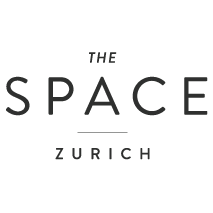## October 2 | New Lunch Class

Tuesdays
12:15 - 13:15 Hanuman Flow (EN)
with Chantal Hauser
at The Space in Zürich | K5
I am very excited to have a lunch class again!

 table div table+table+table+table+table+table+table+table+table+table+table+table+table+table+table+table+table+table+table+table+table+table+table+table+table+table+table+table+table+table+table+table+table+table div table{width:100%;padding:0}table div table+table+table+table+table+table+table+table+table+table+table+table+table+table+table+table+table+table+table+table+table+table+table+table+table+table+table+table+table+table+table+table+table+table div table img{width:96.23%;padding:0;float:none}table div table+table+table+table+table+table+table+table+table+table+table+table+table+table+table+table+table+table+table+table+table+table+table+table+table+table+table+table+table+table+table+table+table+table div table td{width:100%;padding:0 1.88% 18px}/* styles */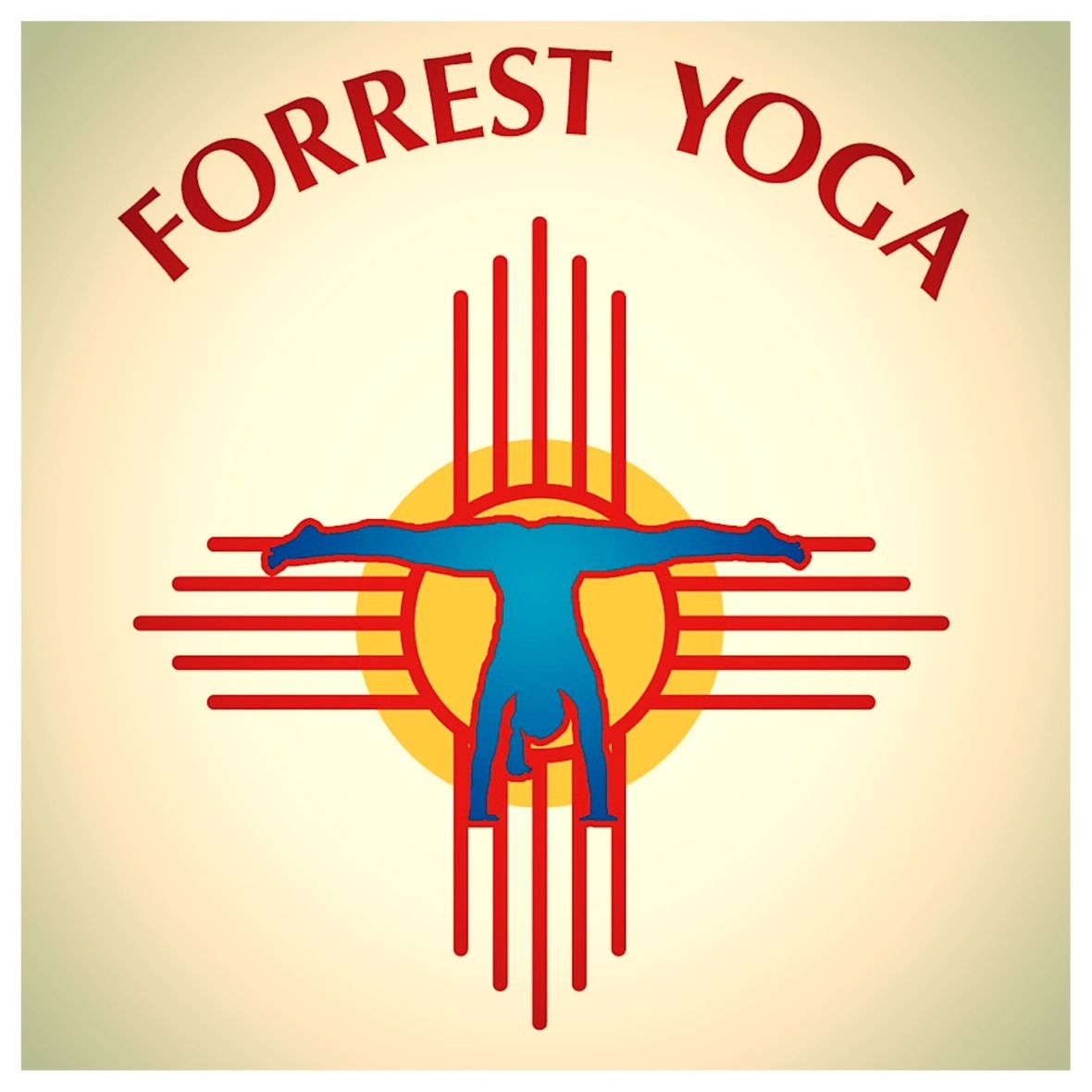## October 4 | Forrest Yoga

Thursday
18:15 - 19:45 Forrest Yoga (EN)
with Chantal Hauser
at Yoga Tribe in Zürich | K3
35.- chf with a Friend | Monkeyship Approved
Come and celebrate my Birthday with me!

 table div table+table+table+table+table+table+table+table+table+table+table+table+table+table+table+table+table+table+table+table+table+table+table+table+table+table+table+table+table+table+table+table+table+table+table+table div table{width:100%;padding:0}table div table+table+table+table+table+table+table+table+table+table+table+table+table+table+table+table+table+table+table+table+table+table+table+table+table+table+table+table+table+table+table+table+table+table+table+table div table img{width:96.23%;padding:0;float:none}table div table+table+table+table+table+table+table+table+table+table+table+table+table+table+table+table+table+table+table+table+table+table+table+table+table+table+table+table+table+table+table+table+table+table+table+table div table td{width:100%;padding:0 1.88% 18px}/* styles */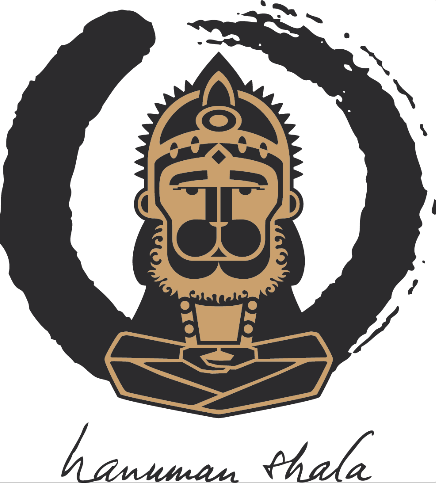## October 6 | Monkey Practice

Sunday
10:00 - 11:45 Monkey Practice (EN)
with Camilla Mariéthoz (Yoga with Camilla)
at The Space in Zürich | K5
35.- chf with a Friend | Monkeyship Approved
Next class with Camilla takes places December 1!

 table div table+table+table+table+table+table+table+table+table+table+table+table+table+table+table+table+table+table+table+table+table+table+table+table+table+table+table+table+table+table+table+table+table+table+table+table+table+table div table{width:100%;padding:0}table div table+table+table+table+table+table+table+table+table+table+table+table+table+table+table+table+table+table+table+table+table+table+table+table+table+table+table+table+table+table+table+table+table+table+table+table+table+table div table img{width:96.23%;padding:0;float:none}table div table+table+table+table+table+table+table+table+table+table+table+table+table+table+table+table+table+table+table+table+table+table+table+table+table+table+table+table+table+table+table+table+table+table+table+table+table+table div table td{width:100%;padding:0 1.88% 18px}/* styles */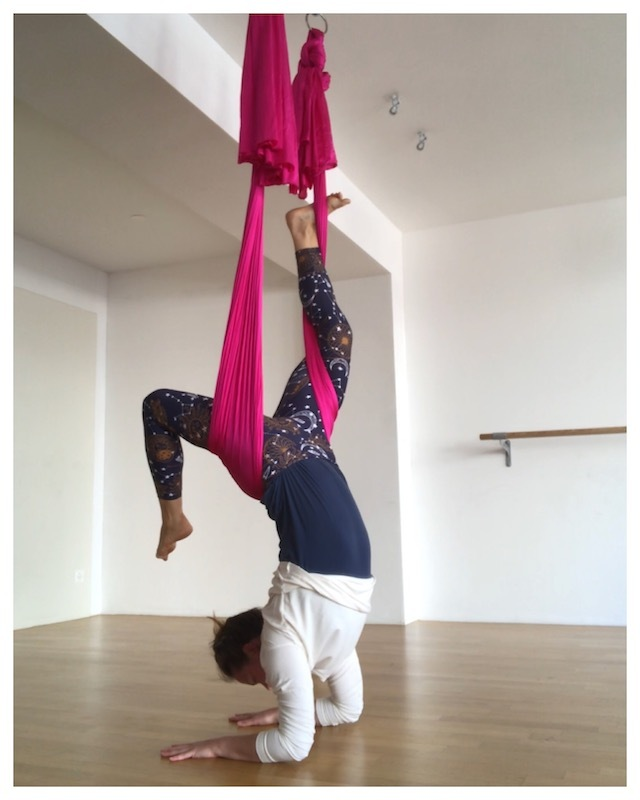## October 7 | Aerial Yoga

Sunday
10:30 - 12:00 Flying Monkey (EN)
with Chantal Hauser
at Power Qi in Zürich | Paradeplatz
50.- chf | Monkeyship Approved +10.-
Next Flying Monkey takes place December 2!

 table div table+table+table+table+table+table+table+table+table+table+table+table+table+table+table+table+table+table+table+table+table+table+table+table+table+table+table+table+table+table+table+table+table+table+table+table+table+table+table+table div table{width:100%;padding:0}table div table+table+table+table+table+table+table+table+table+table+table+table+table+table+table+table+table+table+table+table+table+table+table+table+table+table+table+table+table+table+table+table+table+table+table+table+table+table+table+table div table img{width:96.23%;padding:0;float:none}table div table+table+table+table+table+table+table+table+table+table+table+table+table+table+table+table+table+table+table+table+table+table+table+table+table+table+table+table+table+table+table+table+table+table+table+table+table+table+table+table div table td{width:100%;padding:0 1.88% 18px}/* styles */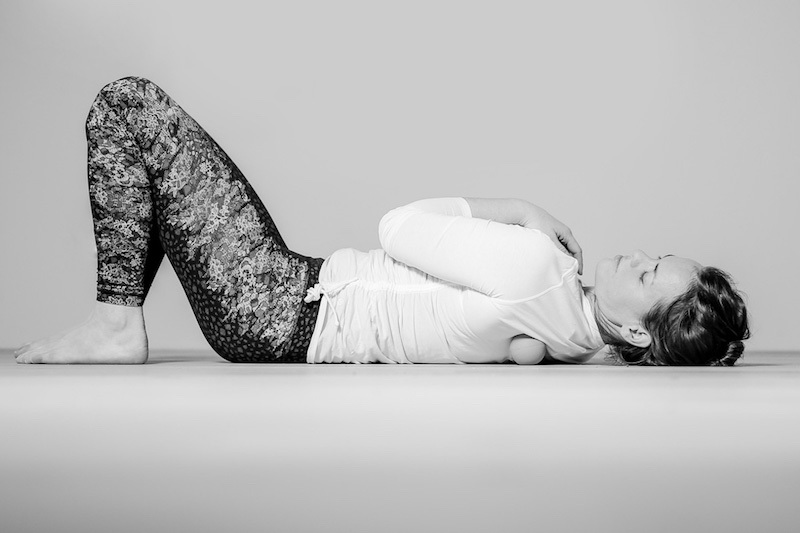## October 21 | Relax + Breathe

Sunday
18:30 - 20:00 Relax + Breathe (EN)
with Chantal Hauser
at Malayoga in Thalwil
35.- chf with a Friend | Monkeyship Approved
Next Dates: 18.11 | 23.12

 table div table+table+table+table+table+table+table+table+table+table+table+table+table+table+table+table+table+table+table+table+table+table+table+table+table+table+table+table+table+table+table+table+table+table+table+table+table+table+table+table+table+table div table{width:100%;padding:0}table div table+table+table+table+table+table+table+table+table+table+table+table+table+table+table+table+table+table+table+table+table+table+table+table+table+table+table+table+table+table+table+table+table+table+table+table+table+table+table+table+table+table div table img{width:96.23%;padding:0;float:none}table div table+table+table+table+table+table+table+table+table+table+table+table+table+table+table+table+table+table+table+table+table+table+table+table+table+table+table+table+table+table+table+table+table+table+table+table+table+table+table+table+table+table div table td{width:100%;padding:0 1.88% 18px}/* styles */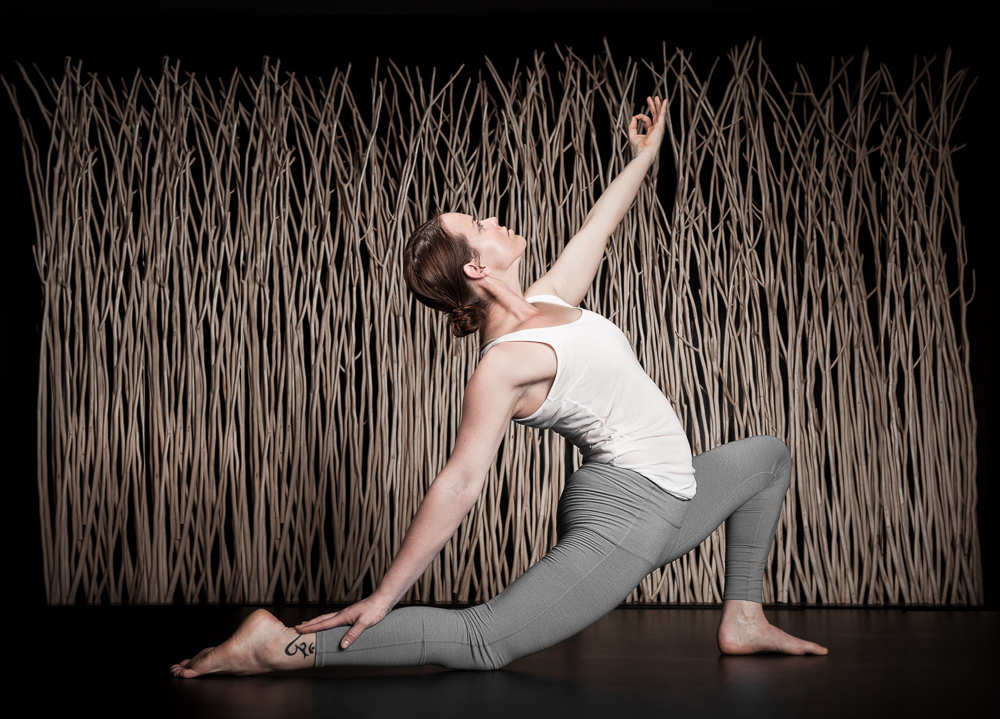## October 28 | Yoga in Kilchberg

Sunday
10:30 - 12:00 Hanuman Flow (EN)
with Chantal Hauser
at Yoga in Kilchberg
Monkeyship Approved | Kilchberg Abo
Next Date: 25.11

 table div table+table+table+table+table+table+table+table+table+table+table+table+table+table+table+table+table+table+table+table+table+table+table+table+table+table+table+table+table+table+table+table+table+table+table+table+table+table+table+table+table+table+table+table div table{width:100%;padding:0}table div table+table+table+table+table+table+table+table+table+table+table+table+table+table+table+table+table+table+table+table+table+table+table+table+table+table+table+table+table+table+table+table+table+table+table+table+table+table+table+table+table+table+table+table div table img{width:96.23%;padding:0;float:none}table div table+table+table+table+table+table+table+table+table+table+table+table+table+table+table+table+table+table+table+table+table+table+table+table+table+table+table+table+table+table+table+table+table+table+table+table+table+table+table+table+table+table+table+table div table td{width:100%;padding:0 1.88% 18px}/* styles */table div table+table+table+table+table+table+table+table+table+table+table+table+table+table+table+table+table+table+table+table+table+table+table+table+table+table+table+table+table+table+table+table+table+table+table+table+table+table+table+table+table+table+table+table+table div table{width:100%;padding:0}table div table+table+table+table+table+table+table+table+table+table+table+table+table+table+table+table+table+table+table+table+table+table+table+table+table+table+table+table+table+table+table+table+table+table+table+table+table+table+table+table+table+table+table+table+table div table img{width:96.23%;padding:0;float:none}table div table+table+table+table+table+table+table+table+table+table+table+table+table+table+table+table+table+table+table+table+table+table+table+table+table+table+table+table+table+table+table+table+table+table+table+table+table+table+table+table+table+table+table+table+table div table td{width:100%;padding:0 1.88% 18px}/* styles */# Forrest Yoga in Hamburg | October 19. + 20. | 2018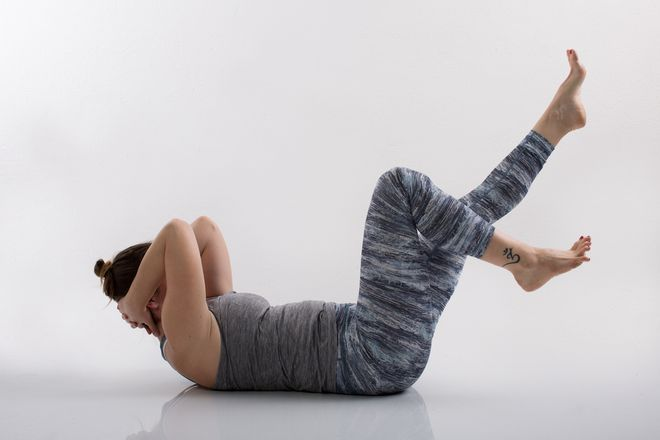19.10.2018
Friday 20:00-22:00
SPACE: Hips, Pelvic Floor & Core
We will work with strengthening your legs and move everything around the waist and the core to find openness and strength in your center. We will also work with the wall and use a few inversions to find comfort and breath while upside down! This class will make you feel grounded and strong. Your hips and pelvic floor will feel spacious and open.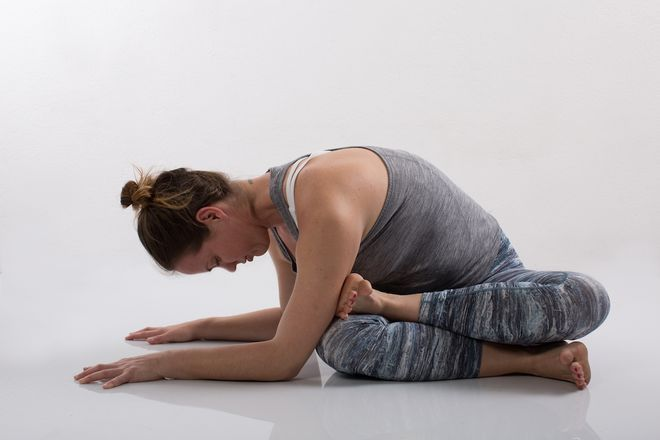20.10.2018
Saturday 15:00-18:00
FREEDOM: Shoulders, Neck, Backbends
Let go of weight and responsibilities and find space in between your shoulder blades. Working with Dolphin variations and incorporating twists will guide us to find freedom in our spine. We will use the wall to find relaxation in the neck and strength in the shoulder area. Deep and healing breath is the foundation for releasing tension all around the upper back, come to this class and leave light and free.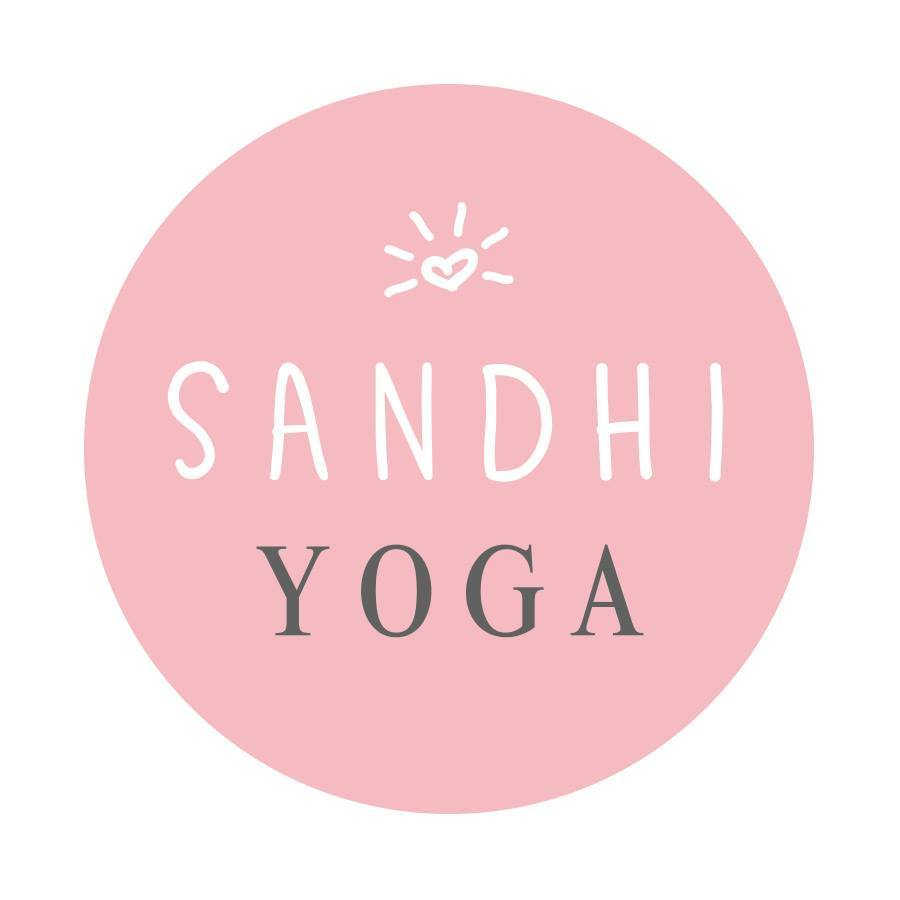at Sandhi Yoga
in Hamburg | Germany

 table div table+table+table+table+table+table+table+table+table+table+table+table+table+table+table+table+table+table+table+table+table+table+table+table+table+table+table+table+table+table+table+table+table+table+table+table+table+table+table+table+table+table+table+table+table+table+table+table+table+table+table+table div table{width:100%;padding:0}table div table+table+table+table+table+table+table+table+table+table+table+table+table+table+table+table+table+table+table+table+table+table+table+table+table+table+table+table+table+table+table+table+table+table+table+table+table+table+table+table+table+table+table+table+table+table+table+table+table+table+table+table div table img{width:96.23%;padding:0;float:none}table div table+table+table+table+table+table+table+table+table+table+table+table+table+table+table+table+table+table+table+table+table+table+table+table+table+table+table+table+table+table+table+table+table+table+table+table+table+table+table+table+table+table+table+table+table+table+table+table+table+table+table+table div table td{width:100%;padding:0 1.88% 18px}/* styles */# Mom + Babe Yoga Course

6x Course | 22.10 - 26.11.2018
Mondays 11:15 - 12:15
at Malayoga in Thalwil
Register Here | 300.- chf | Facebook Event

Connect with your baby and your body after birth. Learn how to move, heal and step into your new role as a Mom! The sessions are primarily for the moms, we will however also incorporate baby massage. Up to crawling age!

 table div table+table+table+table+table+table+table+table+table+table+table+table+table+table+table+table+table+table+table+table+table+table+table+table+table+table+table+table+table+table+table+table+table+table+table+table+table+table+table+table+table+table+table+table+table+table+table+table+table+table+table+table+table+table+table div table{width:100%;padding:0}table div table+table+table+table+table+table+table+table+table+table+table+table+table+table+table+table+table+table+table+table+table+table+table+table+table+table+table+table+table+table+table+table+table+table+table+table+table+table+table+table+table+table+table+table+table+table+table+table+table+table+table+table+table+table+table div table img{width:96.23%;padding:0;float:none}table div table+table+table+table+table+table+table+table+table+table+table+table+table+table+table+table+table+table+table+table+table+table+table+table+table+table+table+table+table+table+table+table+table+table+table+table+table+table+table+table+table+table+table+table+table+table+table+table+table+table+table+table+table+table+table div table td{width:100%;padding:0 1.88% 18px}/* styles */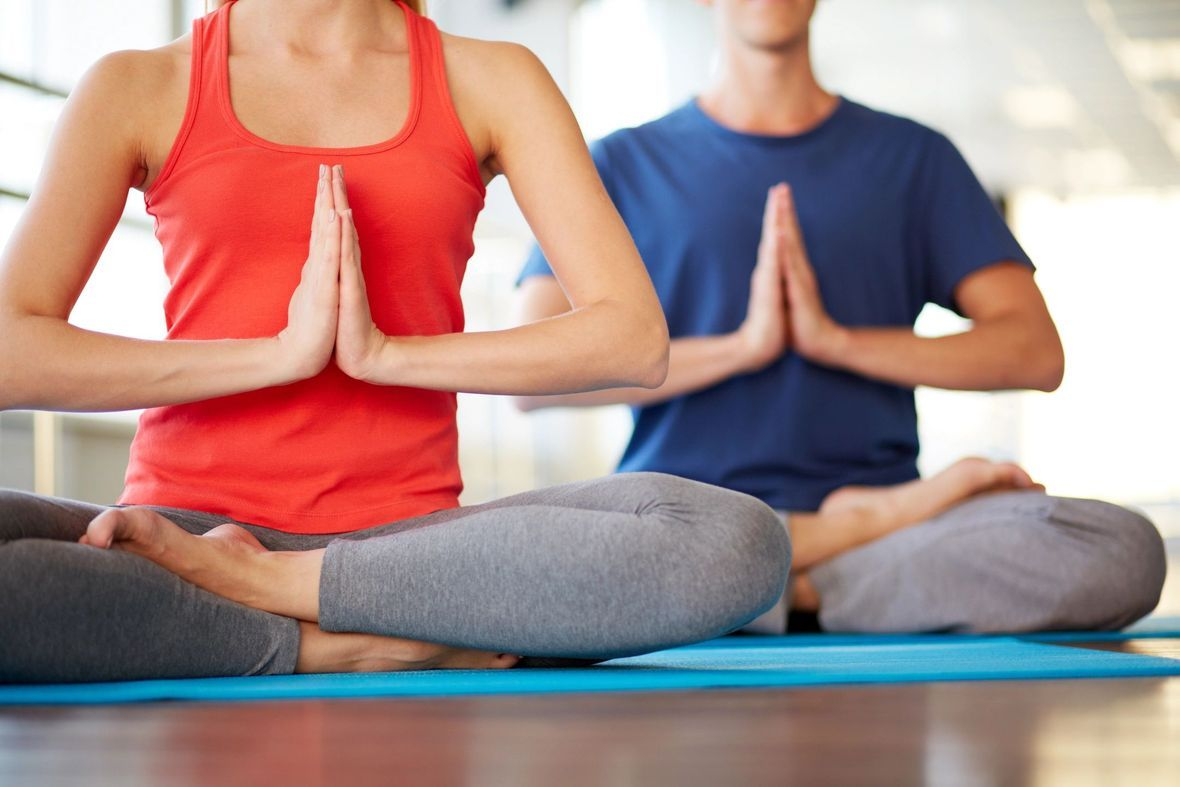# Company Yoga

October 10 + 31
Wednesdays 12:15-13:15
Lunch Yoga (E)
at Yoga Tribe in Zürich | K3
Argus Data Insight People ONLY! Register in Advance

 table div table+table+table+table+table+table+table+table+table+table+table+table+table+table+table+table+table+table+table+table+table+table+table+table+table+table+table+table+table+table+table+table+table+table+table+table+table+table+table+table+table+table+table+table+table+table+table+table+table+table+table+table+table+table+table+table+table div table{width:100%;padding:0}table div table+table+table+table+table+table+table+table+table+table+table+table+table+table+table+table+table+table+table+table+table+table+table+table+table+table+table+table+table+table+table+table+table+table+table+table+table+table+table+table+table+table+table+table+table+table+table+table+table+table+table+table+table+table+table+table+table div table img{width:96.23%;padding:0;float:none}table div table+table+table+table+table+table+table+table+table+table+table+table+table+table+table+table+table+table+table+table+table+table+table+table+table+table+table+table+table+table+table+table+table+table+table+table+table+table+table+table+table+table+table+table+table+table+table+table+table+table+table+table+table+table+table+table+table div table td{width:100%;padding:0 1.88% 18px}/* styles */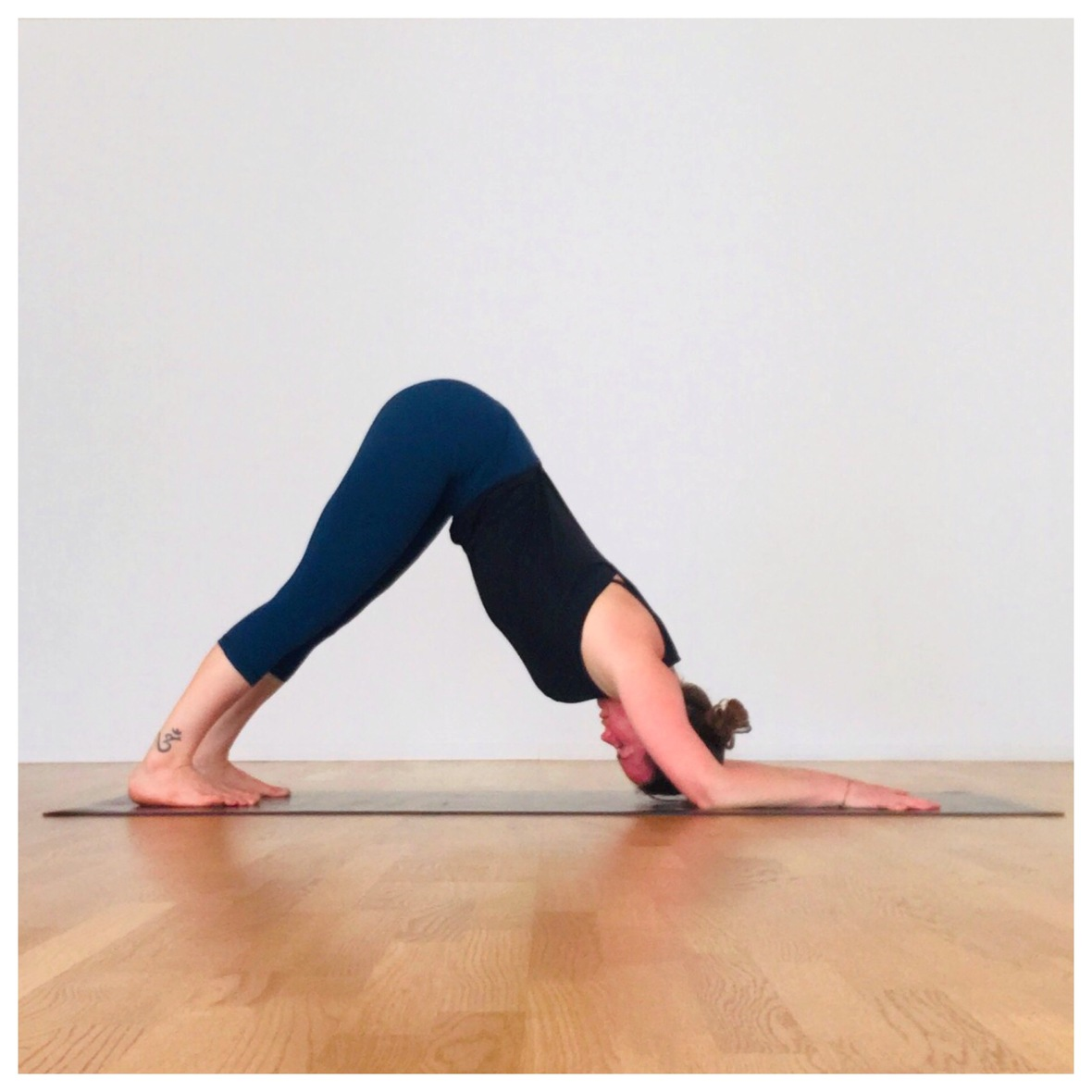# Subs + Extra Classes

October 8
Monday 12:15-13:15
Lunch Yoga (CH)
at Move in Steinhausen
Register Here

 table div table+table+table+table+table+table+table+table+table+table+table+table+table+table+table+table+table+table+table+table+table+table+table+table+table+table+table+table+table+table+table+table+table+table+table+table+table+table+table+table+table+table+table+table+table+table+table+table+table+table+table+table+table+table+table+table+table+table+table div table{width:100%;padding:0}table div table+table+table+table+table+table+table+table+table+table+table+table+table+table+table+table+table+table+table+table+table+table+table+table+table+table+table+table+table+table+table+table+table+table+table+table+table+table+table+table+table+table+table+table+table+table+table+table+table+table+table+table+table+table+table+table+table+table+table div table img{width:96.23%;padding:0;float:none}table div table+table+table+table+table+table+table+table+table+table+table+table+table+table+table+table+table+table+table+table+table+table+table+table+table+table+table+table+table+table+table+table+table+table+table+table+table+table+table+table+table+table+table+table+table+table+table+table+table+table+table+table+table+table+table+table+table+table+table div table td{width:100%;padding:0 1.88% 18px}/* styles *//* styles */ Our October Asana Mash-Up is Utkatasana! It took me a couple of years to feel this pose, to like it and to actually do it correctly! Benefits; the biggest plus of this posture is the balance that it instils. It strengthens all your hamstrings and your ankles. If you are a flexi person, make sure not to hinge over your thighs but to lengthen up! If you are a tighty lift your heels even if it's a bit tricky in the beginning! Variations; feet hip-width apart (like I usually teach and you can see in this picture), you can have your hands together in a traditional hasta mudra or arms open.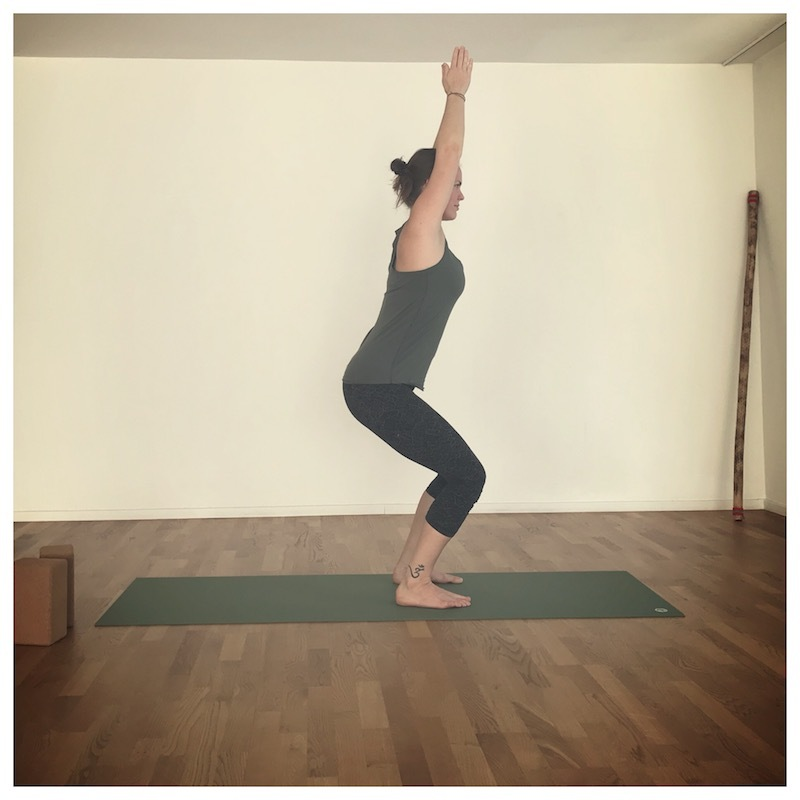Utkatasana - Chair Pose
 table div table+table+table+table+table+table+table+table+table+table+table+table+table+table+table+table+table+table+table+table+table+table+table+table+table+table+table+table+table+table+table+table+table+table+table+table+table+table+table+table+table+table+table+table+table+table+table+table+table+table+table+table+table+table+table+table+table+table+table+table+table+table+table div table{width:100%;padding:0}table div table+table+table+table+table+table+table+table+table+table+table+table+table+table+table+table+table+table+table+table+table+table+table+table+table+table+table+table+table+table+table+table+table+table+table+table+table+table+table+table+table+table+table+table+table+table+table+table+table+table+table+table+table+table+table+table+table+table+table+table+table+table+table div table img{width:96.23%;padding:0;float:none}table div table+table+table+table+table+table+table+table+table+table+table+table+table+table+table+table+table+table+table+table+table+table+table+table+table+table+table+table+table+table+table+table+table+table+table+table+table+table+table+table+table+table+table+table+table+table+table+table+table+table+table+table+table+table+table+table+table+table+table+table+table+table+table div table td{width:100%;padding:0 1.88% 18px}/* styles */Here is a list of 2018 specials that are happening next to my regular classes until the end of the year.

SAVE THE DATE for...

## NOVEMBER

3. + 4.11 Saturday | Sunday | Weggis Yoga Festival
3.11 Forrest Yoga from 17:00 - 18:30 (E)
4.11 Hanuman Flow from 10:30 - 12:00 (E)
at Yoga Meets Weggis in Weggis
Sign Me Up | Facebook Event

10.11 Saturday | Monkey Practice (E)
from 10:00 - 11:45 at The Space in Zürich | Chreis 5
Sign Me Up | Facebook Event

17.11 Saturday | Breath + Inversion Workshop (E)
from 10:00-12:30 | 14:00-16:30 at AgniYoga in Ljubljana | Slovenia
Sign Me Up | Facebook Event

18.11 Sunday | Relax + Breathe (E)
from 18:30 - 20:00 at Malayoga in Thalwil
Sign Me Up | Facebook Event

19.11 Monday | Yoga for Menstrual Cramps (E)
20:00 (Zürich +1GMT) with Holistia Facebook Live Event

24.11 Saturday | The Breathing Project | Thanksgiving Special (E)
from 10:30 - 12:20 at Birdhaus in Zürich | Hürlimann Areal
Sign Me Up

25.11 Sunday | Hanuman Flow (E)
from 10:30 - 12:00 at Yoga in Kilchberg
Sign Me Up | Facebook Event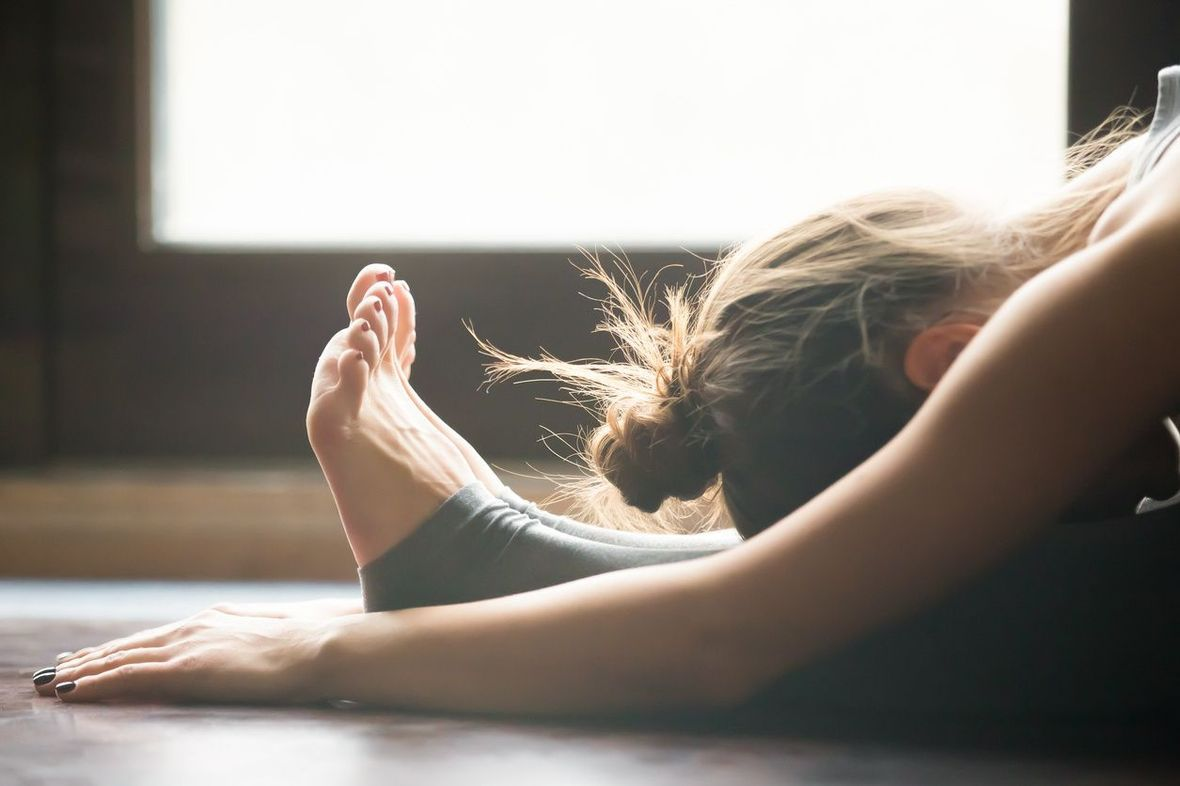We have 3 more months to go Yogis!

## DECEMBER

2.12 Sunday | Flying Monkey (Aerial Yoga) (E)
from 10:30 - 12:00 at Power Yoga Qi in Zürich | Paradeplatz
Sign Me Up

5.12 Wednesday | Charity Yoga Event (E)
from 18:30 - 19:30 at lululemon in Zürich | Dörfli
Sign Me Up

14.12 Friday | Forrest Yoga Intensive (E)
from 18:30 - 20:30 at Body Love Fitness in Zürich | Seefeld
Sign Me Up | Facebook Event

15.12 Saturday | Forrest Yoga Intensive (CH)
from 15:00 - 17:00 at Aloha Yoga in Aarau
Sign Me Up

23.12 Sunday | Relax + Breathe (E)
from 18:30 - 20:00 at Malayoga in Thalwil
Sign Me Up | Facebook Event

27.12 Thursday | Hanuman Flow | After Xmas Special (E)
from 10:00 - 11:15 at Living Yoga in Luzern
Sign Me Up

29.12 Saturday | Creating Core + Intention 2019 (E)
from 10:00 - 11:45 at The Space in Zürich | Chreis 5
Sign Me Up | Facebook Event

I will be teaching a limited schedule during Christmas time. More about my last week of 2018 schedule in the next newsletter!

 table div table+table+table+table+table+table+table+table+table+table+table+table+table+table+table+table+table+table+table+table+table+table+table+table+table+table+table+table+table+table+table+table+table+table+table+table+table+table+table+table+table+table+table+table+table+table+table+table+table+table+table+table+table+table+table+table+table+table+table+table+table+table+table+table+table+table+table+table div table{width:100%;padding:0}table div table+table+table+table+table+table+table+table+table+table+table+table+table+table+table+table+table+table+table+table+table+table+table+table+table+table+table+table+table+table+table+table+table+table+table+table+table+table+table+table+table+table+table+table+table+table+table+table+table+table+table+table+table+table+table+table+table+table+table+table+table+table+table+table+table+table+table+table div table img{width:96.23%;padding:0;float:none}table div table+table+table+table+table+table+table+table+table+table+table+table+table+table+table+table+table+table+table+table+table+table+table+table+table+table+table+table+table+table+table+table+table+table+table+table+table+table+table+table+table+table+table+table+table+table+table+table+table+table+table+table+table+table+table+table+table+table+table+table+table+table+table+table+table+table+table+table div table td{width:100%;padding:0 1.88% 18px}/* styles */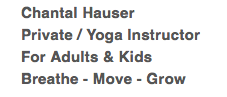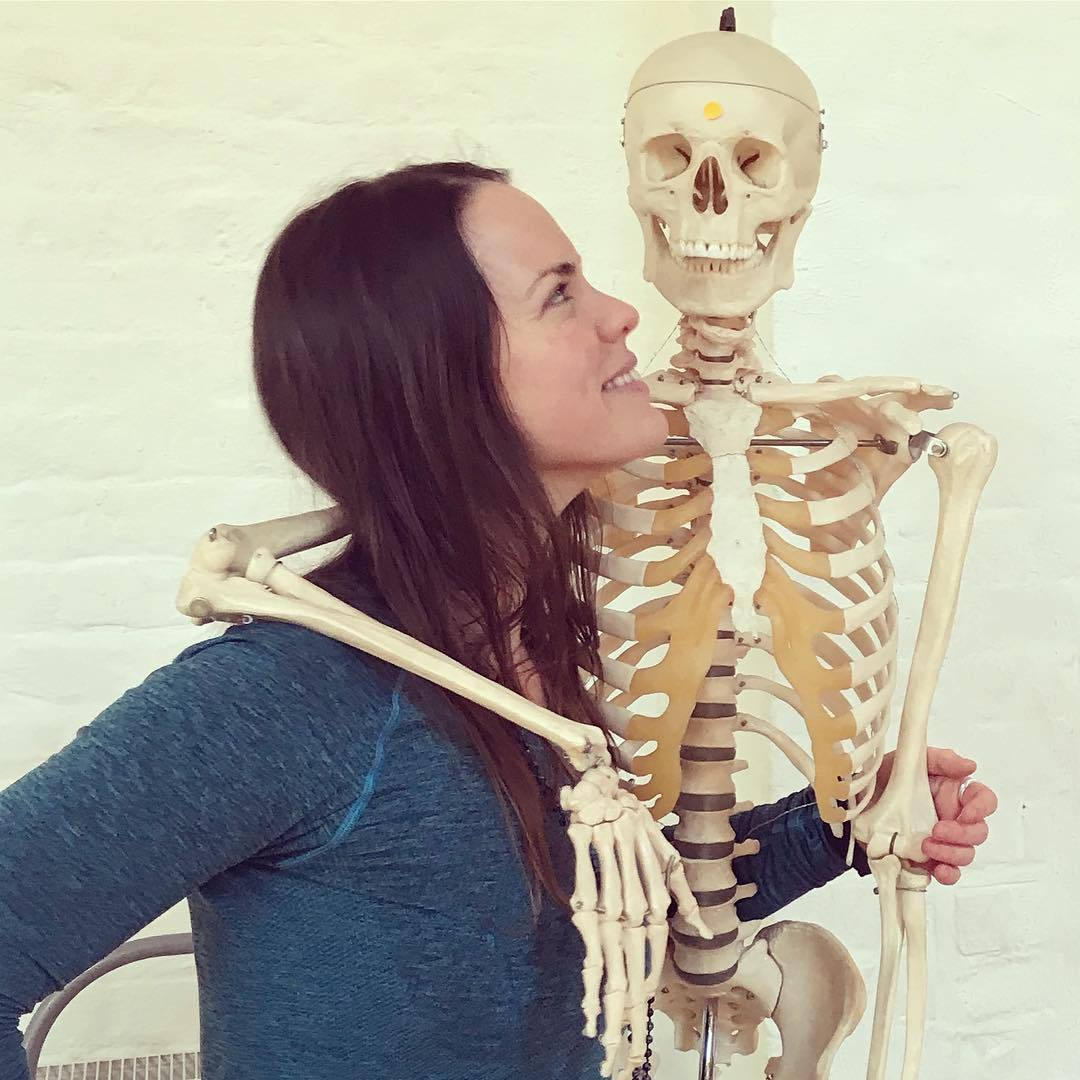table div table+table+table+table+table+table+table+table+table+table+table+table+table+table+table+table+table+table+table+table+table+table+table+table+table+table+table+table+table+table+table+table+table+table+table+table+table+table+table+table+table+table+table+table+table+table+table+table+table+table+table+table+table+table+table+table+table+table+table+table+table+table+table+table+table+table+table+table+table+table+table+table div table{width:100%;padding:0}table div table+table+table+table+table+table+table+table+table+table+table+table+table+table+table+table+table+table+table+table+table+table+table+table+table+table+table+table+table+table+table+table+table+table+table+table+table+table+table+table+table+table+table+table+table+table+table+table+table+table+table+table+table+table+table+table+table+table+table+table+table+table+table+table+table+table+table+table+table+table+table+table div table img{width:96.23%;padding:0;float:none}table div table+table+table+table+table+table+table+table+table+table+table+table+table+table+table+table+table+table+table+table+table+table+table+table+table+table+table+table+table+table+table+table+table+table+table+table+table+table+table+table+table+table+table+table+table+table+table+table+table+table+table+table+table+table+table+table+table+table+table+table+table+table+table+table+table+table+table+table+table+table+table+table div table td{width:100%;padding:0 1.88% 18px}/* styles */Like   Tweet   +1